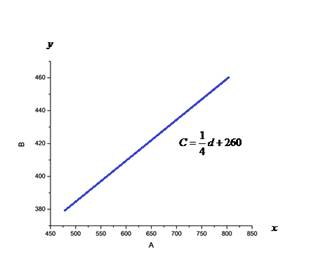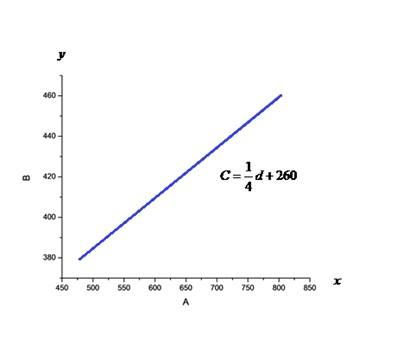# Find a linear equation that relates C and d .### Precalculus: Mathematics for Calcu...

6th Edition
Stewart + 5 others
Publisher: Cengage Learning
ISBN: 9780840068071### Precalculus: Mathematics for Calcu...

6th Edition
Stewart + 5 others
Publisher: Cengage Learning
ISBN: 9780840068071

#### Solutions

Chapter 1.10, Problem 75E

a.

To determine

## Find a linear equation that relates C and d .

Expert Solution

C=14d+260

### Explanation of Solution

Calculation:

The cost of driving 480 mi is $380 for May. The cost of driving 800 mi is$460 for June.

The cost and distance are considered coordinates. The slope of the line is

y2y1x2x1=460380800480=14

So, the line joining the points is

C380=14d120C=14d+260

Where C is the cost of journey and d is the distance travelled.

Hence, the linear equation that relates C and d is C=14d+260

b.

To determine

Expert Solution

Hence, the cost of driving 1500 mi per month is $635 c. To determine ### Draw the graph of the linear equation. What does the slope of the line represent? Expert Solution ## Answer to Problem 75EThe slope represents cost per mile. ### Explanation of Solution Calculation:Note that the cost is represented on yaxis and the distance travelled is represented on xaxis . Hence, the slope represents cost per mile. d. To determine ### What does y−intercept of the graph represents? Expert Solution ## Answer to Problem 75E The yintercept represents the monthly fixed cost. ### Explanation of Solution Calculation: When d=0 , C=$260

which represents the monthly rental spent toward the car.

Hence, the yintercept represents the monthly fixed cost.

e.

To determine

### Why is a linear relationship a suitable model for this situation?

Expert Solution

A linear fuction gives a suitable model because we would expect the cost of driving to be more or less proportional to the number of miles driven.

### Explanation of Solution

Calculation:

A linear fuction gives a suitable model because we would expect the cost of driving to be more or less proportional to the number of miles driven.

Hence, a linear relationship is a suitable model for this situation.

### Have a homework question?

Subscribe to bartleby learn! Ask subject matter experts 30 homework questions each month. Plus, you’ll have access to millions of step-by-step textbook answers!